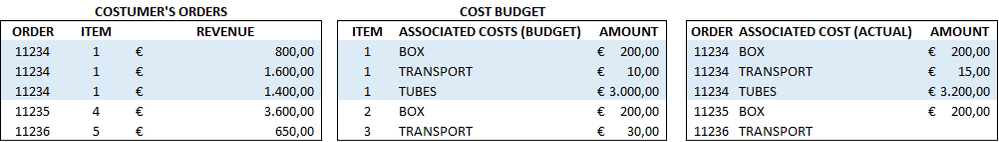cancel
Showing results for
Did you mean:

Fabric is Generally Available. Browse Fabric Presentations. Work towards your Fabric certification with the Cloud Skills Challenge.Helper I

## Summarize problem

Hi,

I've a table with the value of each costumer's order, an order may be divided in additional sub-orders or item according to the type of service required. All items from an order may have the same code like 1 for the 11234 order, or multiple codes like 1, 2, 3 for the 11234 order depending on the supplyer which can be the same or not. In any case each item code is unique to the order so, knowing the item code you can always recognize the order number.

In addition to the revenue, I've also a table with the associated expected costs for each items, constantly updated, and a table with the actual costs which is updated monthly.What I would like to obtain, is a new table which uses the summarize function to summarize, for each order, the actual cost but since this table is uptaded less frequently, I'd also like to see the budget cost in the meantime:

SUMMARIZE('Actual Cost', 'Actual Cost [Order], "Direct Cost", IF(SUM('Actual Cost' [Amount]) = BLANK() || SUM(SUM('Actual Cost' [Amount]) <  SUM('Cost Budget'[Amount]), SUM('Cost Budget'[Amount]) ,(SUM('Actual Cost' [Amount])))

The actual costs are always equal or higher than the expected ones because the budget is from the order confirmation to the supplier while the actual cost is from the purchase invoice which may have additional costs for certificates, assurance, customs duty.

My problem is that when I'tried that formula, the amount from the actual is correct while the budget is three times the real one, like Bi multiplies the value by the numbers of row, since the order is one but the item rows are three. Maybe is because the budget has items and the actual cost table has N. of Order.

I cannot use the RELATED function to create a new column with the order numbers in the budget table because of the many to many relationships.

Can someone help?

Thanks!

1 ACCEPTED SOLUTIONSolution Specialist

Hi, @Luca2020 ,

I suggest that you provide the model relationship of different tables, one to many or many to one, which is helpful to solve this problem.

Nevertheless, I have encountered similar problem before.

My solution is to create a new calculation column in the many-end table to calculate the number of rows that will be repeated after use RELATED() from one-end table, and then your measure can be divided by the value in this column to get a value that won't be repeated.

The formula for this calculate column is :

`column = countrows(RELATEDTABLE(<your main table>))`

Mark this post as solution if this helps, thanks!Solution Specialist

Hi, @Luca2020 ,

I suggest that you provide the model relationship of different tables, one to many or many to one, which is helpful to solve this problem.

Nevertheless, I have encountered similar problem before.

My solution is to create a new calculation column in the many-end table to calculate the number of rows that will be repeated after use RELATED() from one-end table, and then your measure can be divided by the value in this column to get a value that won't be repeated.

The formula for this calculate column is :

`column = countrows(RELATEDTABLE(<your main table>))`

Mark this post as solution if this helps, thanks!Announcements#### Power BI Monthly Update - November 2023

Check out the November 2023 Power BI update to learn about new features.#### Fabric Community News unified experience

Read the latest Fabric Community announcements, including updates on Power BI, Synapse, Data Factory and Data Activator.#### The largest Power BI and Fabric virtual conference

130+ sessions, 130+ speakers, Product managers, MVPs, and experts. All about Power BI and Fabric. Attend online or watch the recordings.Top Solution Authors
Top Kudoed Authors
Users online (4,143)